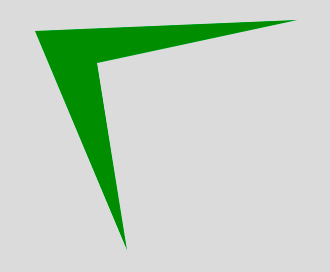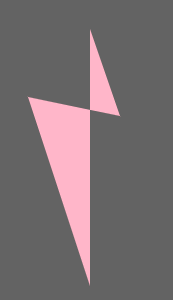• Last Updated : 12 Apr, 2019

The quad() function is an inbuilt function in p5.js which is used to draw a quadrilateral. A quadrilateral is a four-sided polygon. It function creates a shape similar to rectangle, but the angles between two edges are not constrained to 90 degrees.

Syntax:

`quad(x1, y1, x2, y2, x3, y3, x4, y4)`

or

`quad(x1, y1, z1, x2, y2, z2, x3, y3, z3, x4, y4, z4)`

Parameters: This function accepts twelve parameters as mentioned above and described below:

• x1: This parameter takes the x-coordinate of the first point.
• y1: This parameter takes the y-coordinate of the first point.
• z1: This parameter takes the z-coordinate of the first point.
• x2: This parameter takes the x-coordinate of the second point.
• y2: This parameter takes the y-coordinate of the second point.
• z2: This parameter takes the z-coordinate of the second point.
• x3: This parameter takes the x-coordinate of the third point.
• y3: This parameter takes the y-coordinate of the third point.
• z3: This parameter takes the z-coordinate of the third point.
• x4: This parameter takes the z-coordinate of the fourth point.
• y4: This parameter takes the z-coordinate of the fourth point.
• z4: This parameter takes the z-coordinate of the fourth point.

Below programs illustrates the quad() function in P5.js:

Example 1: This example uses quad() function to create a polygon without using z-coordinate.

 `function` `setup() {``     ` `    ``// Create canvas of given size``    ``createCanvas(400, 400);``}``  ` `function` `draw() {``     ` `    ``// Set the background color``    ``background(220);``     ` `    ``noStroke();``     ` `    ``// Set the fill color``    ``fill(``'green'``);``     ` `    ``// x1, y1 = 38, 31; x2, y2 = 300, 20;``    ``// x3, y3 = 100, 63; x4, y4 = 130, 250``    ``quad(38, 31, 300, 20, 100, 63, 130, 250); ``}`

Output:Example 2: This example uses quad() function to create polygon with z-coordinate.

 `function` `setup() {``     ` `    ``// Create canvas of given size``    ``createCanvas(400, 400);``}``  ` `function` `draw() {`` ` `    ``// Set the background color``    ``background(99);``     ` `    ``noStroke();``     ` `    ``// Set the filled color``    ``fill(``'pink'``);``     ` `    ``// x1, y1, z1 = 38, 131, 100;``    ``// x2, y2, z2 = 320, 100, 63; ``    ``// x3, y3, z3 = 130, 150, 134; ``    ``// x4, y4, z4 = 155, 66, 88;``    ``quad(38, 131, 100, 320, 100, 63,``        ``130, 150, 134, 155, 66, 88); ``}`

Output: